WEBINAR
Efficient Green Energy: Exploring Simulation-Driven Design for High-Torque Wind Turbine Machine
Thursday, September 21, 2023
Time
SESSION 1
SESSION 2
CEST (GMT +2)
03:00 PM
08:00 PM
EDT (GMT -4)
09:00 AM
02:00 PM
HOME / Applications / Simulation of high power busbars – Transient Magnetic Analysis inside SOLIDWORKS

# Simulation of high power busbars – Transient Magnetic Analysis inside SOLIDWORKS

Used Tools:## Problem description

In this application, a 2D model of high-power busbars is analyzed using EMWorks2D inside SOLIDWORKS. Transient electromagnetic simulations are performed to compute the different busbars parameters including magnetic field, eddy currents, proximity effects, electromagnetic losses, etc. In addition to these results, several physical phenomena like proximity, skin effects and shielding effect are evaluated. Figures 1a) and 1b) show respectively full 3D model and the simplified 2D model. 2D simplification feature of EMWorks2D is used to convert automatically the initial 3D geometry into 2D surfaces ready for the simulation. The rated current of the simulated busbars is 3kA at 50Hz.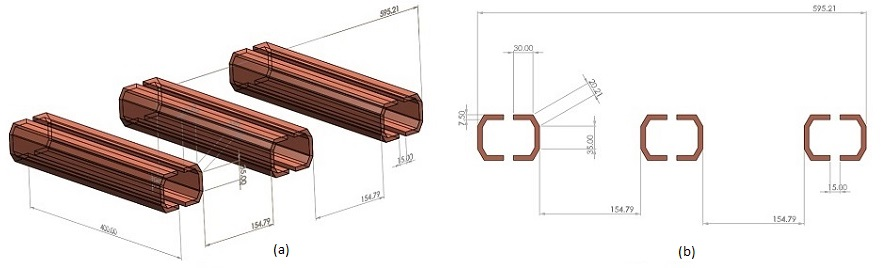Figure 1 - The studied busbars CAD models, a) 3D model, b) 2D model

## Transient magnetic simulation

In this section, transient magnetic analysis is performed to compute different electromagnetic quantities including magnetic field, eddy currents, proximity effects, electromagnetic losses, etc.
Figures 2a) and 2b) illustrates respectively fringe and lines plot of the magnetic flux distribution in the busbars model at different time steps. An animation of the field plot versus time (during two electrical cycles) is shown in Figure 3.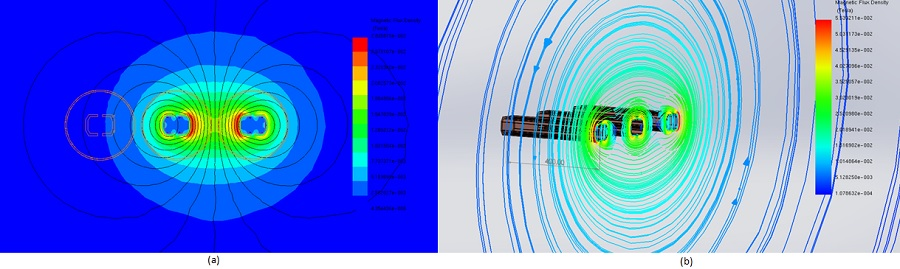Figure 2 - Magnetic field results, a) fringe plot at 0s, b) lines plot at 11ms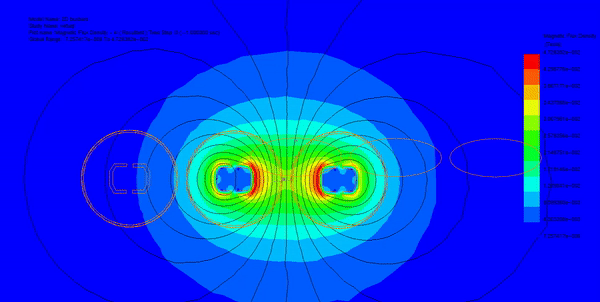Figure 3 - Animation of the magnetic field mapping versus time

Using parametric sweep feature of EMWorks2D, the busbars input currents are varied, and the magnetic field results are evaluated along an axis in XY plane. As can be seen in Figure 4, for the different input currents, the magnetic field has same behavior and it represents few peaks around the busbars conductors.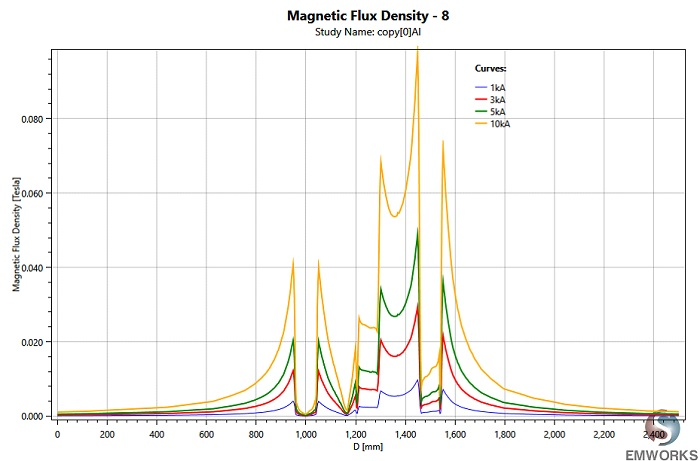Figure 4 -  Magnetic field results between two points

Figure 5 demonstrates the current density distribution of the busbars model phases. Unlike static simulation, in transient simulation the applied current is time varying, different physical phenomena can be observed like skin and proximity effects. It can be seen from Figure 5 that current distribution is not uniform due to the mentioned phenomena. Figure 6 shows the current density through one busbars conductor wall. It decreases by going from one side to another.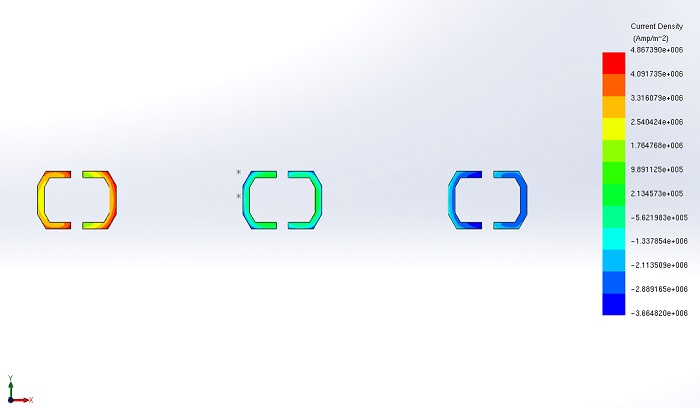Figure 5 -The busbars current distribution at 11ms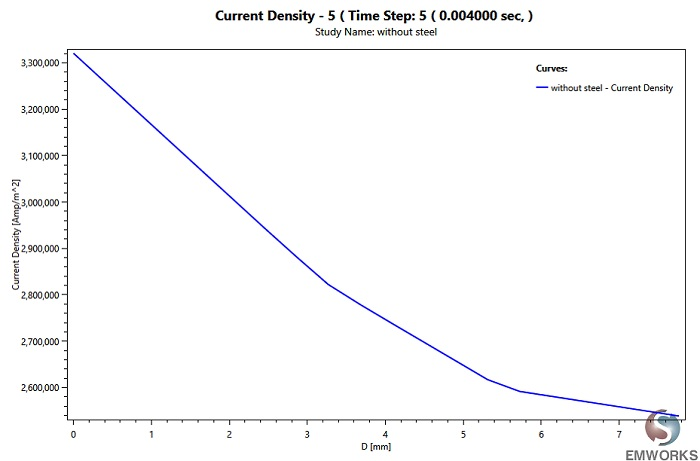Figure 6 - Current density variation along a conductor thickness

The total electromagnetic losses (Figure 7) of the busbar model are computed versus different input currents. The average losses increase linearly with the supplied current as summarized in Figure 8.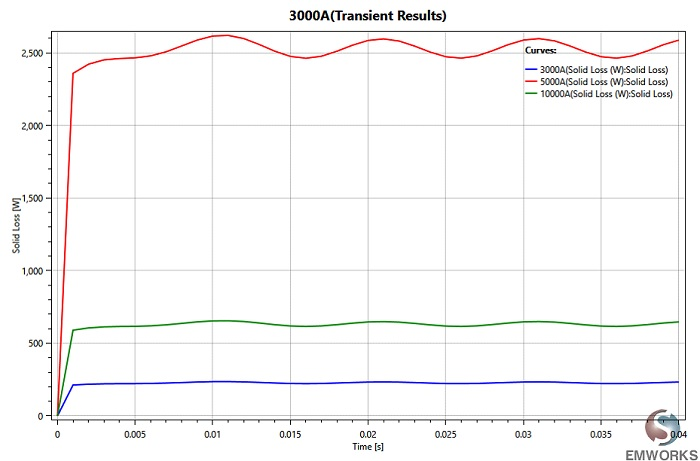Figure 7 - Electromagnetic losses versus time at different input currents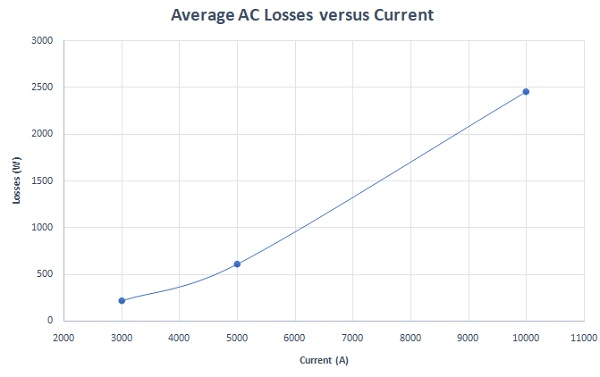Figure 8 -  Averaged AC losses versus current

The busbars are usually made of the copper or aluminum. Therefore, an investigation of the electromagnetic losses in case of using these two materials is required. Figure 9 gives the electromagnetic losses of the studied busbars for both copper and aluminum materials. It shows that aluminum has higher losses than copper and this is due to its higher electrical resistivity. But this doesn’t mean that copper busbars outperforms totally because Aluminum has better weight, cost ratio compared to copper.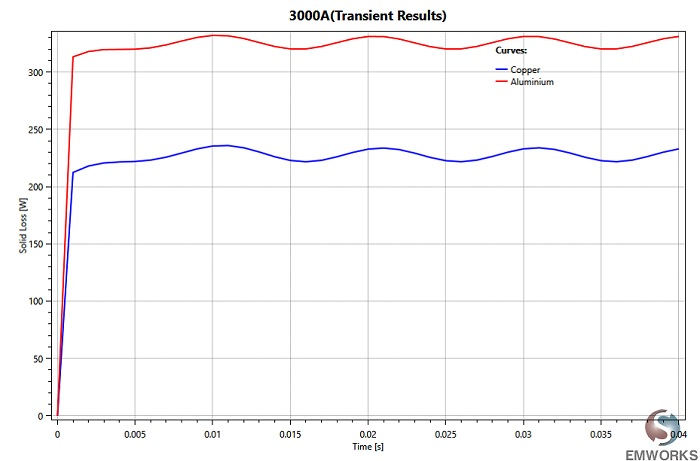Figure 9 - Electromagnetic losses at different materials

Figure 10 contains the total losses of the busbars model versus time at different frequencies. The average losses are maximum at 1kHz (~740W) and minimum when the frequency is 50Hz (~225W) as illustrated in Figure 11. Hence, it can be concluded that the electromagnetic losses increase with the frequency and this is due to skin effect. The skin depth, caused by skin effect phenomenon, decreases with the frequency which makes the busbar resistances and losses bigger. Figure 12 shows also the ratio of AC/DC losses versus frequency. This ratio becomes important with higher frequency. It increases from 1.09 at 50 Hz to reach 3.6 at 1kHz.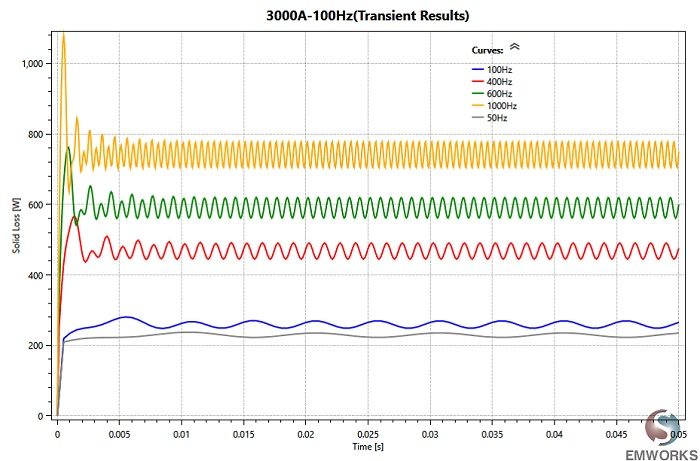Figure 10 - Electromagnetic losses versus frequency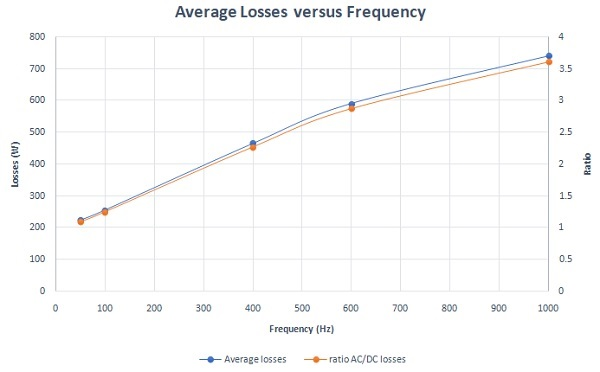Figure 11 -  Average losses and AC/DC losses ratio versus frequency

In Figure 12, the different phases Lorentz force x-components are plotted versus time (the applied current is 3kA peak). It demonstrates that the average Lorentz force of phase 2 is almost null while the total average force is around 4.5N.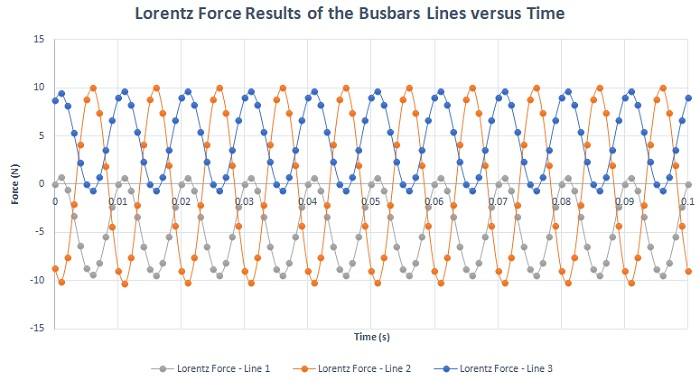Figure 12 - Lorentz force x-components results of the busbars three phases

## Shielding Effects

Figures 14 and 15 contain, respectively, a comparison of static and time-varying magnetic field in case with/ without metallic shielding. In both cases and unlike unshielded busbars, the magnetic field distribution becomes smaller in term of magnitude and limited in small area around the busbars when the steel shielding is added.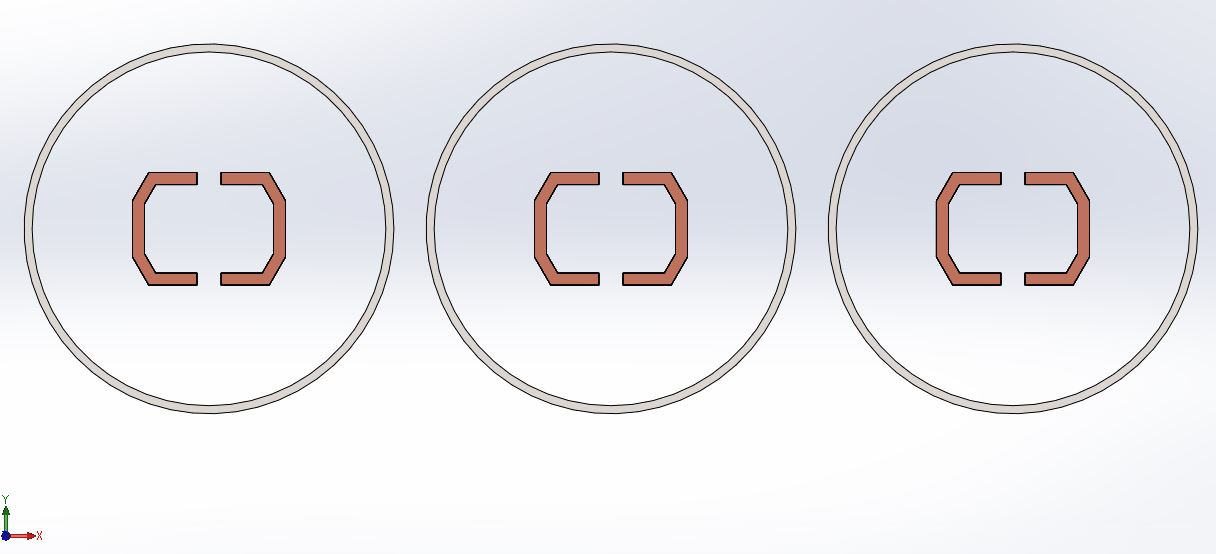Figure 13 - Shielded busbars model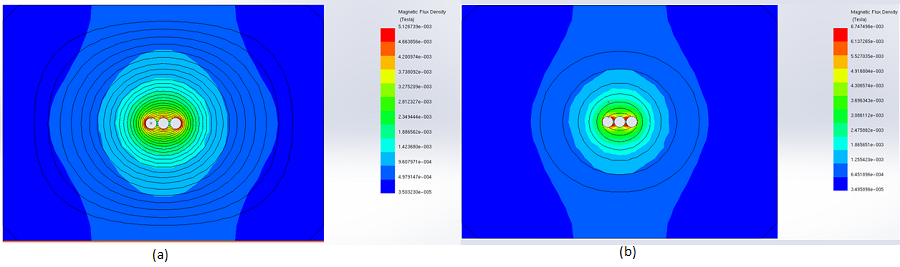Figure 14 - Static magnetic field results in the surrounding air region, a) without metallic shielding, b) with metallic shielding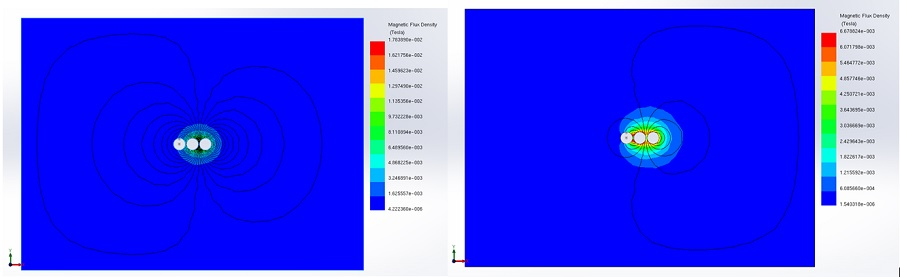Figure 15 - AC magnetic field results in the surrounding air region, a) without metallic shielding, b) with metallic shielding

However, shielding ensures the protection of electronic components and human health against electromagnetic radiation, but it results in additional power loss. Figure below shows the total busbars losses when it is surrounded by metallic shield.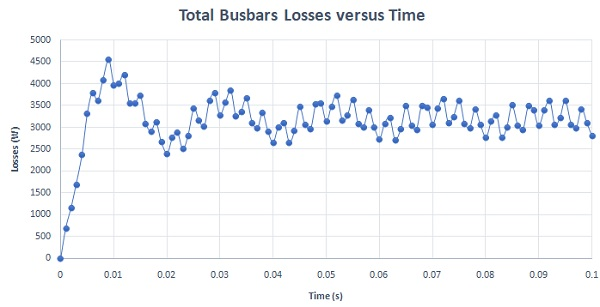Figure 16 -  Electromagnetic loss in case of steel shielded busbars

Below are the Lorentz force results of the three busbars lines in case of metallic shielding. As can be seen, overall, the average forces are less than 1N compared to 4.5N in case of unshielded busbars. Hence, the steel shielding can reduce the impact of magnetic force especially in case of short circuit.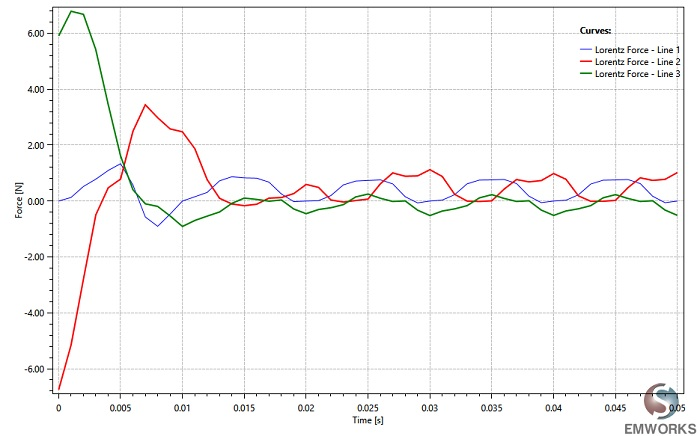Figure 17 - Lorentz force results in the busbars three phases in case of metallic shielding

## Short Circuit Response

Figure 18 shows a comparison of Lorentz force results in the third phase of the busbars. This comparison demonstrates that the Lorentz force is reduced in presence of the metallic shielding.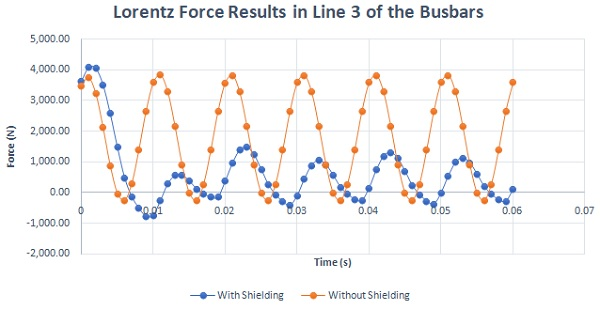Figure 18 - Lorentz force results under short circuit condition

Figure 19 shows the electromagnetic losses under short circuit condition in case of shielded and unshielded busbars model. The short circuit losses are considerably huge and may cause the damage of the system.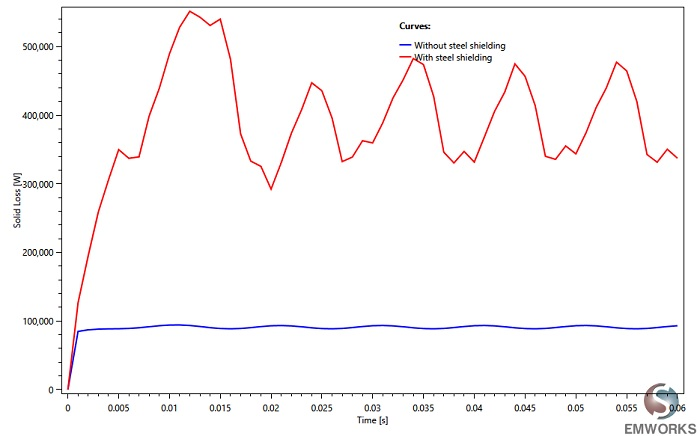Figure 19 - Electromagnetic losses under short circuit condition

## Summary

In this application note, EMWorks2D was used to study a busbar model. Transient magnetic analysis was performed to compute different output results of the simulated busbars under several operation conditions. Magnetic fields, electromagnetic losses, circuit parameters were calculated using EMWorks2D.